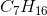# SAT II Chemistry : Molar Mass

## Example Questions

### Example Question #1 : Molar Mass

What is the approximate percentage of carbon by mass in heptane, which has the molecular formula?Explanation:

First, calculate the molar mass of heptane, which isWe can calculate the percentage of carbon by mass in heptane by creating a fraction of the mass of carbon over the mass of the entire molecule and converting it into a percentage:For every 100 g of heptane, 84 g must come from carbon. This means that the percentage of carbon by mass is